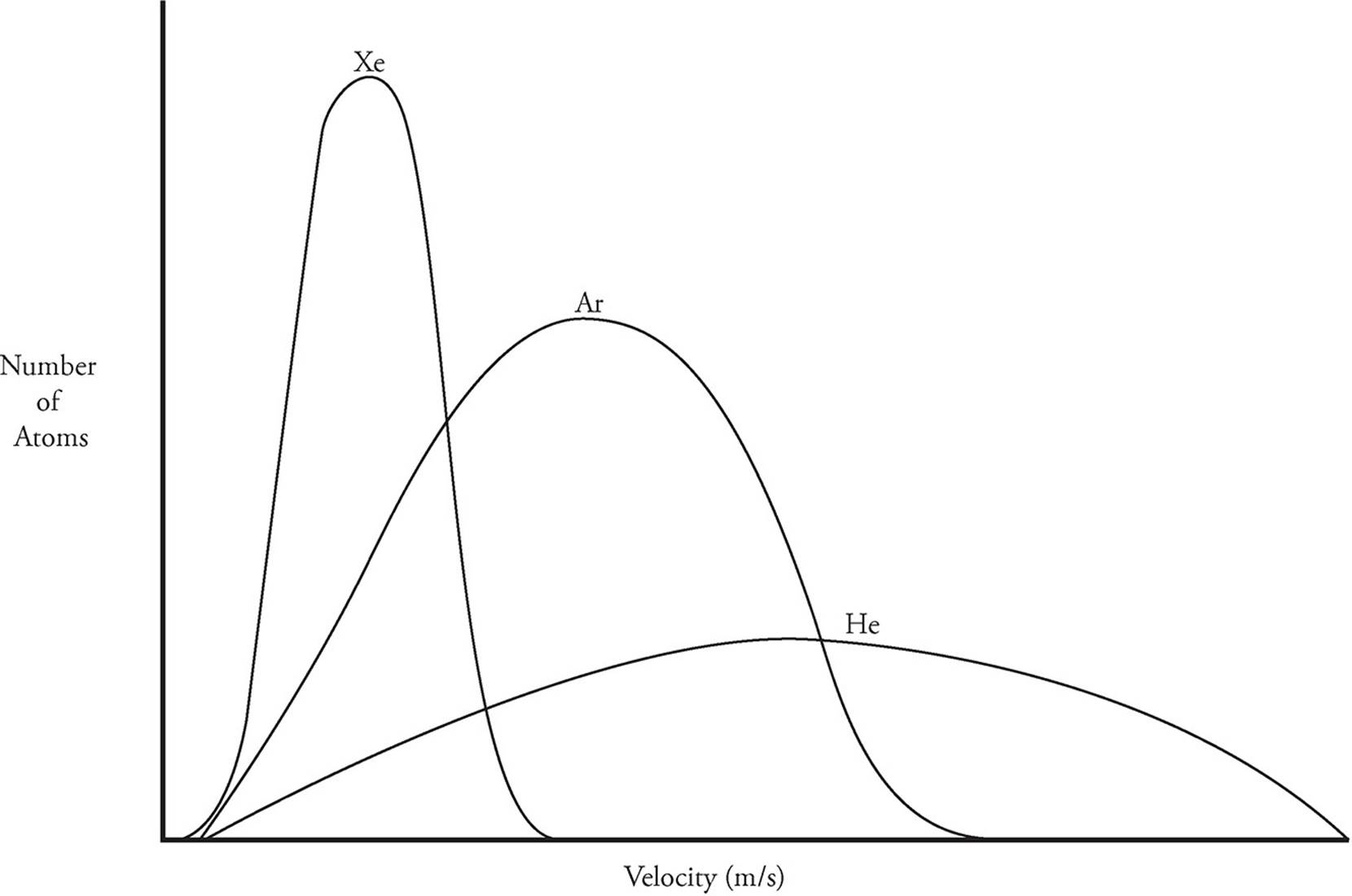﻿ ﻿MAXWELL-BOLTZMANN DIAGRAMS - Bonding and Phases - Content Review for the AP Chemistry Exam - Cracking the AP Chemistry Exam

## Cracking the AP Chemistry Exam

Part IV

Content Review for the AP Chemistry Exam

Chapter 4

Big Idea #2: Bonding and Phases

MAXWELL-BOLTZMANN DIAGRAMS

A Maxwell-Boltzmann diagram shows the range of velocities that the molecules of a gas can be found at. Molecules at a given temperature are not all moving at the same velocity. When determining the temperature, we take the average velocity of all the molecules and use that in the relevant equation to calculate temperature. You do not need to know that equation (unless you are taking AP Physics!). All you need to know here is that temperature is directly proportional to kinetic energy.

The first type of Maxwell-Boltzmann diagram involves plotting the velocity distributions for the molecules of one particular gas at multiple temperatures. In the following diagram, there are three curves representing a sample of nitrogen gas at 100 K, 300 K, and 500 K.As you can see, the higher the temperature of the gas, the larger the range is for the velocities of the individual molecules. Gases at higher temperatures have greater kinetic energy (KE), and as all the molecules in this example have the same mass, the increased KE is due to the increased velocity of the gas molecules.

Maxwell-Boltzmann diagrams are also used to show a number of different gases at the same temperature. The following diagram shows helium, argon, and xenon gas, all at 300 K:In this case, all of the gases have the same amount of total kinetic energy because they have identical temperatures. However, not all of the atoms have the same mass. If the atoms have smaller masses, they must have greater velocities in order to have a kinetic energy identical to that of atoms with greater mass. Because helium atoms have the least mass, they have the highest average velocity. Xenon atoms, which have a much greater mass, have a correspondingly lower velocity.

﻿